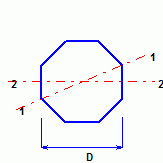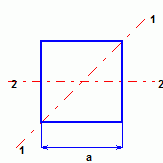HomeGeneral Tools
-------------------------------------------------------------------------------------------------------------------------------------------------------------------------------------------------------------------------------------------
Design Criteria for Vertical Equipment
Design Criteria for Vertical Equipment

Basic diameter = diameter O.D. + 2 (shell thickness + insulation),
effective projected area = height x I.F. x basic diameter.

For shape factor, rough surface is used.

Gust factor > 2.0, for slender vessel, vortex shedding shall be considered.

Intensification Factor (I.F.):

Diameter <= 760 mm, I.F. = 1.50
Diameter 900 to 1350 mm, I.F. = 1.40
Diameter 1370 to 1960 mm, I.F. = 1.30
Diameter 1980 to 2570 mm, I.F. = 1.20
Diameter >= 2590 mm, I.F. = 1.18

For tall vessel (>= 30 m), the strong wind 1/100 year wind pressure is used.

Operating Load + Temperature + Wind (Seismic)
Test + 0.5 Wind (Seismic)

3. Anchor Bolts Design:

Maximum tension = 4.M / (N x BC) - W / N.

M - maximum overturning moment at base of vessel.
N - number of bolts.
BC - diameter of bolt circle.
W - weight of vessel.

Normally, for bearing type bolt shear capacity, only half of anchors actually transfer the shear load.

If anchor bolt in tension and concrete pull-out capacity is not enough, vertical dowels are required to transfer tensile forces to foundations, for development length of rebars , check ACI351.3R 4.2.1

4. Pedestal Design:

Pedestal shall be designed as cantilever columns, k = 2.0.

The pedestal size <= 1.5m, square pedestal may be economical, normally, octagonal shape is used.

Top of pedestal is 300 above grade.

Face to face pedestal size:

Bolt circle + 200 mm
Bolt circle + 8 x bolt diameters.
Bolt circle + Sleeve diameter + 150 mm
Diameter of base plate + 100 mm.

Maximum dowel tension = 4.Mu / (n x BD) - Wu / n.

Mu - maximum overturning moment at base of pedestal.
n - number of bars.
BD - diameter of bar circle.
Wu - Factored vertical load (factor =0.85).

Minimum reinforcement:

Octagon <= 2.7 m, 16 - 25M vertical with 10M ties at 400 mm maximum.
Octagon 2.75 to 3.7m, 24 - 20M vertical with 15M ties at 250 mm maximum.
Octagon > 3.7 m, 32 - 20M vertical with 15M ties at 250 mm maximum.
Pedestal over 1.8m shall have a mesh at the top, 10M at 200mm spacing in both direction, minimum.

5. Footing Design:

The footing size < 1.5m, square footing may be economical, normally, octagonal shape is used.
Minimum thickness is 300 mm.
Minimum rebars 15M @ 300 c/c for top and bottom both direction.
Top of footing shall be minimum 300 mm above grade.

6. Normally, the stability ratio is = 1.5 for sliding and overturning, but for tall vessel (>= 30m), 2.0 is used.

7. Soil Bearing:

Allowable soil bearing pressure may be increased 33% for wind or seismic loading.

Bearing pressure for square footing:

For axis 1-1:
B.P.max = [ 1 + 8.485e / a] P /a2
B.P.min = [ 1 - 8.485e / a] P /a2

For axis 2-2:
e <= a/6     B.P.max = P/a [1 + 6e/a]     B.P.min = P/a [1 - 6e/a]
e > a/6     B.P. = 2P / [3a(a/2 - e)]

Bearing pressure for octagonal footing:

B.P.max = 1.2[ 1+ 8.19 e/D] P/ D2
B.P.min = 1.2[ 1- 8.19 e/D] P/ D2

8. Foundamental Frequency:

For a vessel with constant wall thickness, constant diameter, and a fixed base, the natural frequencies are those for a cantilever beam:

ni = ki / H2[ E.I /M]0.5

ni = frequency of mode i (Hertz)

Ki = constant

= 0.56 for mode 1
= 3.51 for mode 2
= 9.82 for mode 3
= 19.2 for mode 4

H = height of vessel(m)
E = Modulus of elasticity (pa)
I = moment of inertia of vessel(m4),   I = p.d3.t / 8
M = mass of vessel per unit length. (kg per meter or N.s2 /m per meter, = N / 9.81)
d = inside vessel diameter (m)
t = vessel wall thickness (m)

9. Tall vessel structural damping:

It depends not only on the vessel itself, but also on the vessel soil-structure interaction.

Concrete vessel: 0.0150 – 0.025
Steel vessel: 0.005 – 0.015
Unlined steel stack: 0.0016 – 0.006
Gunite-lined steel stack: 0.003 – 0.012
Concrete chimney: 0.004 – 0.020

The lower values are appropriate for foundation on rock or piles, average values are appropriate for foundations on compacted soil,
higher values are appropriate for vessels supported by elevated structures or soft soils.

10. Period for seismic design

Reference: ASCE Guilde for Seismic Evaluation and Design of Petrochemical Facilities 4.A-4

T = 7.78x10-6 (H / D)2 [ 12 W. D / t ]0.5

H - vessel height (ft.)
W - vessel linear weight (lb/ft.)
t - vessel shell thickness (in.)
D - vessel shell outside diameter (ft.)
T - period (sec.)